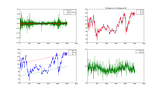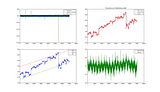# Stock mechanics and the simple harmonic oscillator

This entry is part of 15 in the series Grails Finance

Grails Finance 1.5

The simple harmonic oscillator models an ideal physical system, without friction, for which a constant force/acceleration results in a constant displacement or the other way around. A typical example would be a mass on a spring. Anyway this model could possibly be used as a very rough first order approximation for your usual stock market, as stock prices tend to go up and down around an equilibrium. Of course, as one says in common parlance, it would be stupid to put too much faith in this model.

## Exporting to CSV

Numpy is really great and together with Matplotlib, it is incredibly powerful. So I decided to go for a Numpy centric approach. This meant that I needed to export the data from my Grails database. I messed around with the Grails export plugin, but it seemed easier to just do it in my own way. I added this code in the HistoricalQueryService

```1 2 3 4 5 6 7 8 9 10 11 12 13 14 15 16 17 18 19 20 21 22 23 24 25 26 27 28 ... def queryVals2Recs(symbol, fieldNames) { def records = new TreeMap() def instrument = Instrument.findBySymbol(symbol)   def firstField = Field.findByName(fieldNames) def firstFieldVals = FieldValue.findAllByInstrumentAndField(instrument, firstField) firstFieldVals = firstFieldVals.sort { a, b -> a.added <=> b.added }   DateTimeFormatter fmt = DateTimeFormat.forPattern("yyyy-MM-dd 00:00:00");     firstFieldVals.each { def dateString = fmt.print(it.added) def dayValues = [dateString]   for(i in 0..<fieldNames.size()) { def fval = FieldValue.findAll("from FieldValue fv where fv.instrument = :ins and fv.field.name = :fn and fv.added = :a", [ins: instrument, fn: fieldNames[i], a: it.added] ) dayValues << fval.val }   records[dateString] = dayValues.flatten() }   return records } ...```

On the controller side I just print comma separated values and make the data downloadable in CSV format.

```1 2 3 4 5 6 7 8 9 10 11 12 13 14 15 16 ... if(params.symbol) { def recs = historicalQueryService.queryVals2Recs(params.symbol, ["Open", "High", "Low", "Close", "Volume"]) response.contentType = ConfigurationHolder.config.grails.mime.types["csv"] response.setHeader("Content-disposition", "attachment; filename=" + params.symbol + ".csv")   recs.each { key,value -> response.outputStream << value.join(',') response.outputStream << "\n" }   response.outputStream.flush() response.outputStream.close() } } ...```

## Numpy analysis

I calculated the “spring” constant of the Dow Jones stocks with the exported end of day data. From the constant I get the oscillation period. Based on initial conditions I deduced a sine wave. The code below plots several moving averages using daily and “monthly” periods. For convenience, I define a month to have 22 days. Also I calculate a line fit by taking a point from each monthly cycle. This leads to top and bottom bands by adding and subtracting 1 standard deviation from the fit.

```1 2 3 4 5 6 7 8 9 10 11 12 13 14 15 16 17 18 19 20 21 22 23 24 25 26 27 28 29 30 31 32 33 34 35 36 37 38 39 40 41 42 43 44 45 46 47 48 49 50 51 52 53 54 55 56 57 58 59 60 61 62 63 64 65 66 67 68 69 70 71 72 73 74 75 76 77 78 #!/usr/bin/env python from numpy import * from pylab import * from matplotlib.font_manager import FontProperties   o,h,l,c,v = loadtxt('IBM.csv', delimiter=',', usecols=(1,2,3,4,5), unpack=True)   relchange = diff(c)/c[:len(c)-1]   def calc_k(relchange, arr): diffs2 = diff(relchange,n=2) ks = []   for i in range(0, len(arr)): if arr[i] != 0: ks.append(diffs2[i]/arr[i])   return abs(average(ks))   K = calc_k(relchange, relchange[2:]) T = 2 * pi / sqrt(K) A = relchange[int(T/4)]   t = arange(0, len(c), 1) wave = A * sin( sqrt(K) * t[1:])     font0 = FontProperties() font0.set_size('small')   def ema(arr, T): avg = copy(arr)   for i in range(T,len(arr)): avg[i] = ((T-1) * avg[i-1] + arr[i])/T   return avg   indices22 = arange(0,len(c), 22) c22 = take(c, indices22) relchange22 = diff(c22)/c22[:len(c22)-1] K22 = calc_k(relchange22, relchange22[2:]) T22 = 2 * pi * sqrt(K22) / K22   subplot(221) p1=plot(t[1:],wave) p2=plot(t[1:],relchange) p3=plot(t[1:], ema(relchange, int(T) ) ) legend([p1,p2,p3], ['Harmonic','Relative Change', 'MA T=' + str(int(T))], prop=font0)   movingAvg = ema(c, int(T)) movingAvg22 = ema(c, int(T22 * 22))   subplot(222) title('T(1days)=' + str( int(T) ) + ' T(22days)=' + str( int(T22 * 22))) p1=plot(t,movingAvg) p2=plot(t,movingAvg22) p3=plot(t,c) legend([p1,p2,p3], ['MA ' + str(int(T)),'MA ' + str(int(T22 * 22)), 'Close'], prop=font0)   subplot(224) p1=semilogy(t, ema(v, int(T22 * 22) ) ) p2=semilogy(t, v) legend([p1,p2], ['MA ' + str(int(T22 * 22)), 'Volume'], prop=font0)   indicesTops = arange(0, len(c), int(T22 * 22)) tops = take(c, indicesTops) A = vstack([indicesTops, ones(len(indicesTops))]).T aTop, bTop = linalg.lstsq(A, tops)   sigma = std(c)   subplot(223) p1=plot(t,c) p2=plot(t, aTop * t + bTop - sigma) p3=plot(t, aTop * t + bTop + sigma) legend([p1,p2, p3], ['Close','Bottom', 'Top'], prop=font0) show()```

And the periods are in.

In the table below are plots for IBM end of day data and intraday data 1 min bar data.

 EOD Intraday